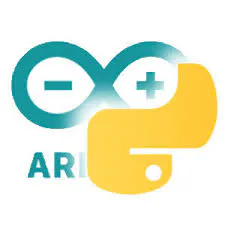# Pix-a-Sketch - A Virtual Etch-a-Sketch on an LED Matrix

Use two rotary encoders to draw whatever picture your heart desires, and then shake it to erase the image — just like the real thing!

IntermediateFull instructions provided6 hours6,376

## Things used in this project

### Hardware components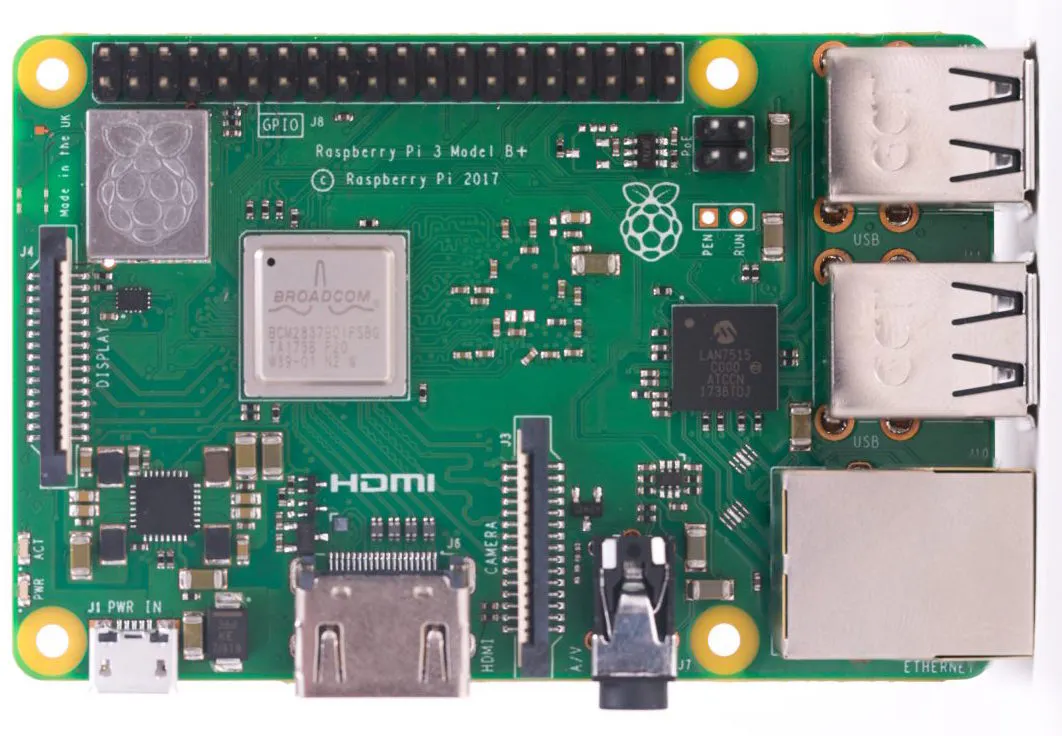Raspberry Pi 3 Model B+
×1
×1
 DFRobot 64 x 64 RGB LED Matrix Panel
×1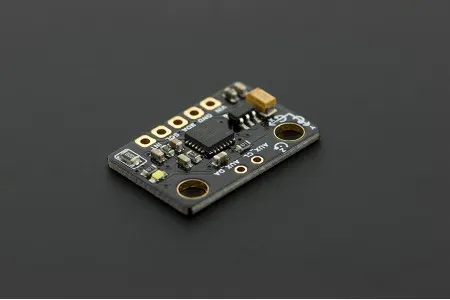DFRobot 6 DOF Sensor - MPU6050
×1Arduino Nano R3
×1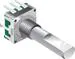Rotary Encoder with Push-Button
×2
 Momentary Pushbutton
×1

### Software apps and online servicesMicrosoft VS CodeArduino IDE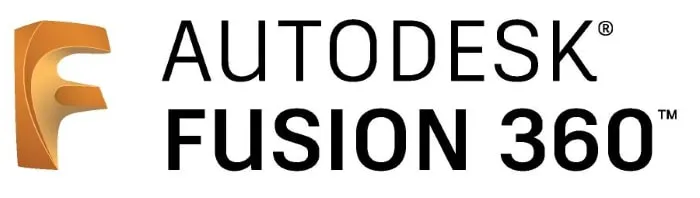Autodesk Fusion 360

### Hand tools and fabrication machines3D Printer (generic)Soldering iron (generic)
 CNC Router

## Custom parts and enclosures

### Plastic Body

Split the model to print if necessary

### Base

Cut with CNC router

## Schematics

### Schematic for Encoder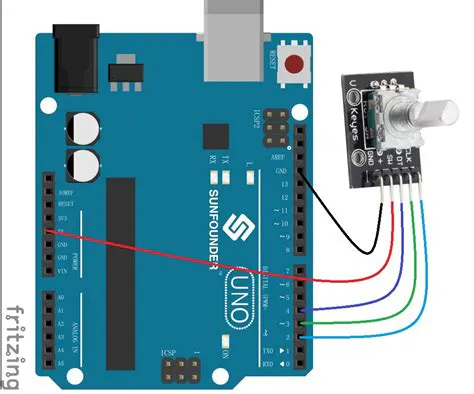## Code

### Raspberry Pi Code

Python
```import smbus
import sys
from rgbmatrix import RGBMatrix, RGBMatrixOptions, graphics
from PIL import Image, ImageDraw
from mpu6050 import mpu6050
from gpiozero import Button
from time import sleep
import random

def constrain(x, a, b):
if x < a:
return a
elif x > b:
return b
else:
return x

class PixelSketch(object):
BTN_PIN = 25
SHAKE_THRESHOLD = 4.0   # shake harder than 6 m/s^2 to clear
def __init__(self, interrupt_en = 0, matrix_w = 64, matrix_h = 64):
self.matrix_w = matrix_w
self.matrix_h = matrix_h
self.options = RGBMatrixOptions()
self.options.rows = matrix_w
self.options.cols = matrix_h
self.options.chain_length = 1
self.options.parallel = 1
self.matrix = RGBMatrix(options=self.options)
self.matrix.Clear()
self.bus = self.sensor.bus
self.bus.write_byte_data(PixelSketch.ARDUINO_ADDRESS, 0x00, 0x01)   # reset the Arduino
self.bus.write_byte_data(PixelSketch.ARDUINO_ADDRESS, 0x01, interrupt_en)   # enable interrupt
self.button = Button(PixelSketch.BTN_PIN, bounce_time = .1, hold_time = 2.0)
self.button.when_held = self.resetBoard
self.cursorPosition = [0, 0]
self.pixelMap = [[0 for x in range(matrix_w)] for y in range(matrix_h)]     # keep track of matrix in memory
self.litPixels = []
def update(self):
goalPos = [self.cursorPosition + encoderAmts, self.cursorPosition + encoderAmts]
goalPos = constrain(goalPos, 0, self.matrix_w-1)
goalPos = constrain(goalPos, 0, self.matrix_h-1)
stepAmts = [0, 0]
if encoderAmts != 0:
stepAmts = int(encoderAmts / abs(encoderAmts))
if encoderAmts != 0:
stepAmts = int(encoderAmts / abs(encoderAmts))
shouldContinue = True
while shouldContinue:
prevPos = [self.cursorPosition, self.cursorPosition]
self.matrix.SetPixel(prevPos, prevPos, 150, 150, 0)
if self.cursorPosition == goalPos:
shouldContinue = False
else:
self.cursorPosition += stepAmts
self.pixelMap[self.cursorPosition][self.cursorPosition] = 1
self.litPixels.append([self.cursorPosition, self.cursorPosition])
self.matrix.SetPixel(self.cursorPosition, self.cursorPosition, 150, 150, 0)
self.matrix.SetPixel(prevPos, prevPos, 0, 0, 150)
prevPos = [self.cursorPosition, self.cursorPosition]
shouldContinue = True
if self.cursorPosition == goalPos:
shouldContinue = False
else:
self.cursorPosition += stepAmts
self.pixelMap[self.cursorPosition][self.cursorPosition] = 1
self.litPixels.append([self.cursorPosition, self.cursorPosition])
self.matrix.SetPixel(self.cursorPosition, self.cursorPosition, 150, 150, 0)
self.matrix.SetPixel(prevPos, prevPos, 0, 0, 150)
shouldContinue = True
if self.isBeingShaken():
for x in range(5):
if len(self.litPixels) > 0:
randChoice = random.choice(self.litPixels)
self.pixelMap[randChoice][randChoice] = 0
self.matrix.SetPixel(randChoice, randChoice, 0, 0, 0)
self.litPixels.remove(randChoice)
sleep(.005)
sleep(0.1)

def isBeingShaken(self):
vals = self.sensor.get_accel_data()
print(vals)
if abs(vals['x']) >= PixelSketch.SHAKE_THRESHOLD or abs(vals['y']) >= PixelSketch.SHAKE_THRESHOLD:
return True
return False

encoderValues = [0, 0]
try:
print(encoderValues)
for index, val in enumerate(encoderValues):
if val > 127:
encoderValues[index] = (256 - val) * -1
except OSError:
pass
encoderValues = -encoderValues
encoderValues = -encoderValues
return encoderValues

def resetBoard(self):
self.litPixels = []
self.cursorPosition = [0, 0]
self.pixelMap = [[0 for x in range(self.matrix_w)] for y in range(self.matrix_h)]
self.bus.write_byte_data(PixelSketch.ARDUINO_ADDRESS, 0x00, 0x01)   # reset the Arduino
self.matrix.Clear()

if __name__ == "__main__":
sketch = PixelSketch()
while 1:
sketch.update()
```

### Arduino Nano Code

C/C++
```#include <Wire.h>
#include "EncoderStepCounter.h"

#define ENCODER_PINL1 2
#define ENCODER_PINL2 3
#define ENCODER_PINR1 4
#define ENCODER_PINR2 5

#define OP_RESET 0x00
#define OP_INTERRUPT_EN 0x01

volatile uint8_t opcode = 0x04;
int8_t registerStack;

volatile int8_t posL = 0, posR = 0;

EncoderStepCounter encoderL(2, 3);
EncoderStepCounter encoderR(4, 5);

void setup() {
Serial.begin(115200);
delay(50);
Serial.println("It works");
Wire.onRequest(requestEvent);
encoderL.begin();
encoderR.begin();
softReset();
}

void loop() {
encoderL.tick();
encoderR.tick();

int8_t pos = encoderL.getPosition();
if(pos != 0) {
posL += pos;
Serial.print("L: "); Serial.println(posL);
encoderL.reset();
}
pos = encoderR.getPosition();
if(pos != 0) {
posR += pos;
Serial.print("R: "); Serial.println(posR);
encoderR.reset();
}
}

if(bytes > 1) {
switch(opcode) {
case OP_RESET:
break;
case OP_INTERRUPT_EN:
break;
}
}
}

void requestEvent() {
switch(opcode) {
Wire.write(posL);
posL = 0;
break;
Wire.write(posR);
posR = 0;
break;
}
}

void softReset() {
encoderL.reset();
encoderR.reset();
opcode = 0x04;
}
```

## Credits

### Evan Rust

113 projects • 1001 followers
IoT, web, and embedded systems enthusiast. Contact me for product reviews, custom project requests, or contract roles.